# Aug 16 2018 Intensely edgy cat with FluidFFT

This is a reimplementaion of Patrick Bos's notebook which showcases xtensor-fftw. Here, we use fluidfft instead.

## Setup¶

In :
import fluidfft     # fft, ifft and other operators

import numpy as np  # numpy arrays!

# for displaying images
from PIL import Image
import urllib
import io
import matplotlib.pyplot as plt

In :
# We go one step further and directly load from the internet
%matplotlib inline

fd = urllib.request.urlopen(url)
im = Image.open(image_file)
im = np.array(im, dtype=np.float64)  # Convert the PIL.PngImagePlugin.PngImageFile object to a numpy array
return im

def display(im):
plt.imshow(im, cmap="gist_gray")
plt.axis('off')


## Intense cat¶

Let's perform some Fourier space operations on this public domain, intense kitty:

In :
image = load_image("https://upload.wikimedia.org/wikipedia/commons/6/67/Intensity_image_with_gradient_images.png")
display(image)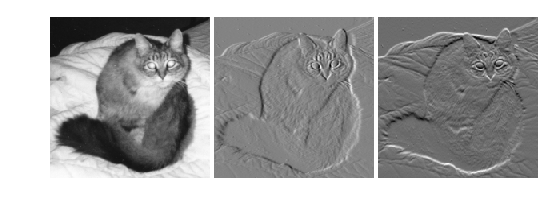In :
image = image[5:-5, 5:205]  # Crop to the first frame and trim the frame edges
display(image)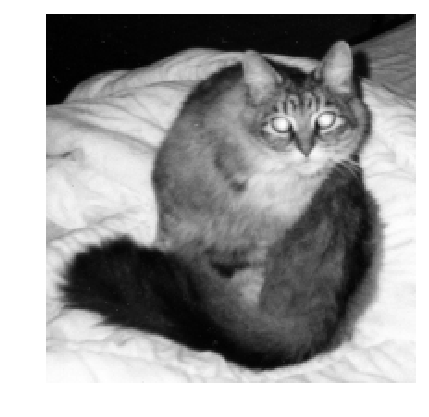## Edge detection on intensity image¶

Unlike in the original notebook wherein,

We're going to use FFT to do some simple edge detection. To simplify, we do this on the black and white "intensity" image. In this case, the kitty is already black and white, but in fact it's still encoded in three RGB channels in the PNG file. Combining these, in general, could be done as follows ...

we skip it, since PIL was kind enough to do it for us:

In :
image.shape

Out:
(200, 200)
In :
image_bw = image


Next, we transform to Fourier space using fluidfft's real FFT transform. We have to rely on the fft2d.with_pyfftw backend since the other Cythonized fft2d.with_fftw2d implementation assumes a periodic boundary.

In :
# Prepare our FFT object
o = fluidfft.create_fft_object("fft2d.with_pyfftw", *image.shape)  # A more powerful option is to use an "Operator" class, demonstrated below

In :
image_fs_bw = o.fft(image_bw)


The simplest way to do some edge detection is by calculating the derivative of the intensity image. The derivative (the rate of change) is high where a sharp transition from low to high intensity occurs between two pixels, close to zero when there is little change and highly negative for high to low transition.

The derivative of an image can be calculated by multiplying the Fourier transform of the image by $\sqrt{-1} \boldsymbol{k} = i \boldsymbol{k}$ and then transforming the result back to real space. This must be done in each direction and then the results can be combined to get the magnitude of the gradient, which is a good multi-directional proxy for both kinds of edges (intensity transitions form low to high and from high to low).

fluidfft's operator classes also supplies these wavenumbers $\boldsymbol{k}$, so you do not need to build them :).

In :
from fluidfft.fft2d.operators import OperatorsPseudoSpectral2D

op = OperatorsPseudoSpectral2D(*image.shape, lx=np.pi, ly=np.pi, fft="fft2d.with_pyfftw")  # The lengths are arbitary

In :
kx = op.KX
ky = op.KY # N.B.: we use broadcasting to multiply in the right direction

In :
# do both derivatives separately
d_image_dx_fs_bw = 1j * kx * image_fs_bw
d_image_dy_fs_bw = 1j * ky * image_fs_bw


An effortless and efficent way to do the gradient calculation was to use the Pythranized function.

In :
d_image_dx_fs_bw, d_image_dy_fs_bw = op.gradfft_from_fft(image_fs_bw)

In :
# transform back to normal space
d_image_dx_bw = op.ifft(d_image_dx_fs_bw)
d_image_dy_bw = op.ifft(d_image_dy_fs_bw)

In :
# and square-sum them in real space to get the gradient magnitude
d_image_grad_bw = np.sqrt(d_image_dx_bw ** 2 + d_image_dy_bw ** 2)

In :
display(d_image_grad_bw)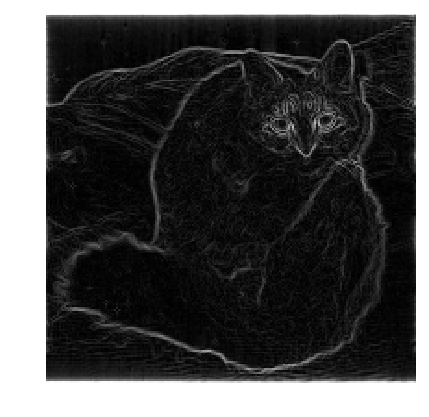To get maximum contrast, rescale so that the maximum is 255 (the maximum brightness value, i.e. bright white):

In :
amax_d_image_grad_bw = d_image_grad_bw.max()

In :
display(d_image_grad_bw / amax_d_image_grad_bw * 255)## Rescaling¶

To inspect the separate horizontal and vertical components, we need to rescale the range of derivative values so that they all fit into the [0,255] range of the RGB space. We subtract the minimum to set negative values to zero and then divide by (max-min) and multiply by 255 to set the maximum to 255 (and scale all intermediate values accordingly).

We can then also sum the both components to get a slightly different perspective on the above "absolute" multi-directional edge detector.

In :
d_image_dx_bw_rescale = 255 * (d_image_dx_bw - d_image_dx_bw.min()) / (d_image_dx_bw.max() - d_image_dx_bw.min())
d_image_dy_bw_rescale = 255 * (d_image_dy_bw - d_image_dy_bw.min()) / (d_image_dy_bw.max() - d_image_dy_bw.min())

d_image_grad_bw_rescale = np.sqrt(d_image_dx_bw_rescale * d_image_dx_bw_rescale + d_image_dy_bw_rescale * d_image_dy_bw_rescale);


### Horizontal¶

In :
display(d_image_dx_bw_rescale)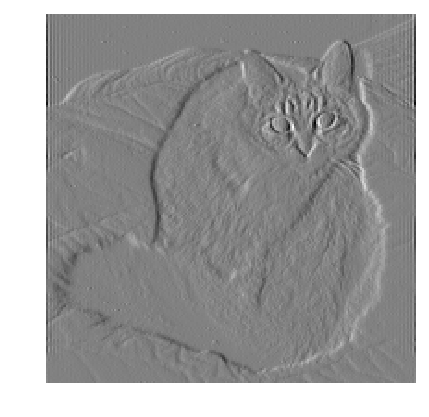### Vertical¶

In :
display(d_image_dy_bw_rescale)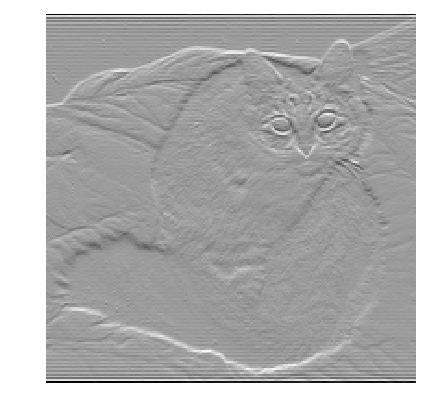The result differs slightly from that in the original Wikipedia image, which is because their gradient function is a bit different. Their matrix gradient method smoothes the image a bit, leading to slightly less sharp edges, but also less sensitivity to noise in the image.

### Combined¶

In :
display(d_image_grad_bw_rescale)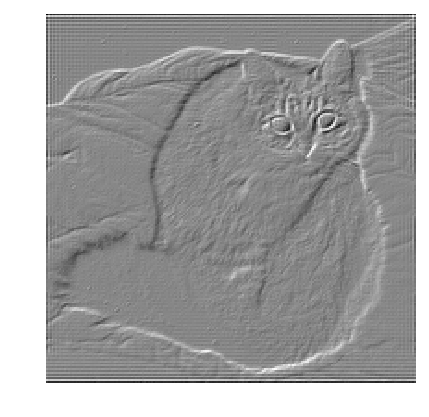This modified post was written entirely in the Jupyter notebook. You can download this notebook, or see a static view on nbviewer.